Home
Hostname: page-component-55b6f6c457-b6fb2 Total loading time: 0.167 Render date: 2021-09-28T15:10:34.088Z Has data issue: true Feature Flags: { "shouldUseShareProductTool": true, "shouldUseHypothesis": true, "isUnsiloEnabled": true, "metricsAbstractViews": false, "figures": true, "newCiteModal": false, "newCitedByModal": true, "newEcommerce": true, "newUsageEvents": true }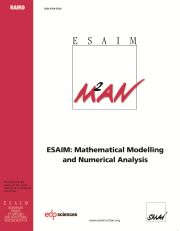ESAIM: Mathematical Modelling and Numerical Analysis

# Sparse finite element approximation of high-dimensional transport-dominated diffusion problems

Published online by Cambridge University Press:  30 July 2008

## Abstract

We develop the analysis of stabilized sparse tensor-product finite element methods for high-dimensional, non-self-adjoint and possibly degenerate second-order partial differential equations of the form $-a:\nabla\nabla u + b \cdot \nabla u + cu = f(x)$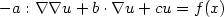, $x \in \Omega = (0,1)^d \subset \mathbb{R}^d$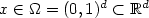, where $a \in \mathbb{R}^{d\times d}$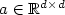is a symmetric positive semidefinite matrix, using piecewise polynomials of degree p ≥ 1. Our convergence analysis is based on new high-dimensional approximation results in sparse tensor-product spaces. We show that the error between the analytical solution u and its stabilized sparse finite element approximation u h on a partition of Ω of mesh size h = hL = 2-L satisfies the following bound in the streamline-diffusion norm $|||\cdot|||_{\rm SD}$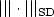, provided u belongs to the space $\mathcal{H}^{k+1}(\Omega)$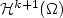of functions with square-integrable mixed (k+1)st derivatives: $|||u-u_h|||_{\rm SD}\leq C_{p,t} d^2 \max\{(2-p)_+,\kappa_0^{d-1},\kappa_1^d\} (|\sqrt{a}| h_L^t + |b|^{\frac{1}{2}} h_L^{t+\frac{1}{2}} + c^{\frac{1}{2}} h_L^{t+1} \!)|u|_{\mathcal{H}^{t+1}(\Omega)}, \qquad \qquad \qquad$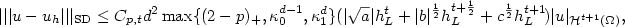where $\kappa_i=\kappa_i(p,t,L)$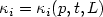, i=0,1, and $1 \leq t \leq \min(k,p)$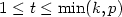. We show, under various mild conditions relating L to p, L to d, or p to d, that in the case of elliptic transport-dominated diffusion problems $\kappa_0, \kappa_1 \in (0,1)$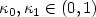, and hence for p ≥ 1 the 'error constant' $C_{p,t} d^2 \max\{(2-p)_+,\kappa_0^{d-1},\kappa_1^d\}$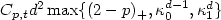exhibits exponential decay as d → ∞; in the case of a general symmetric positive semidefinite matrix a, the error constant is shown to grow no faster than $\mathcal{O}(d^2)$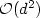. In any case, in the absence of assumptions that relate L, p and d, the error $|||u - u_h|||_{\rm SD}$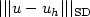is still bounded by $\kappa_\ast^{d-1} |\log_2 h_L|^{d-1}\mathcal{O}(|\sqrt{a}| h_L^t + |b|^{\frac{1}{2}} h_L^{t+\frac{1}{2}} + c^{\frac{1}{2}} h_L^{t+1})$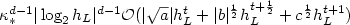, where $\kappa_\ast \in (0,1)$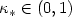for all L, p, d ≥ 2.

Type
Research Article
Information

## Access options

Get access to the full version of this content by using one of the access options below. (Log in options will check for institutional or personal access. Content may require purchase if you do not have access.)

## References

Babenko, K., Approximation by trigonometric polynomials is a certain class of periodic functions of several variables. Soviet Math. Dokl. 1 (1960) 672675. Russian original in Dokl. Akad. Nauk SSSR 132 (1960) 982–985.
Barrett, J.W. and Süli, E., Existence of global weak solutions to kinetic models of dilute polymers. Multiscale Model. Simul. 6 (2007) 506546. CrossRef
Barrett, J.W., Schwab, C. and Süli, E., Existence of global weak solutions for some polymeric flow models. Math. Models Methods Appl. Sci. 15 (2005) 939983. CrossRef
R.F. Bass, Diffusion and Elliptic Operators. Springer-Verlag, New York (1997).
T.S. Blyth and E.F. Robertson, Further Linear Algebra. Springer-Verlag, London (2002).
H.-J. Bungartz, Finite elements of higher order on sparse grids. Habilitation thesis, Informatik, TU München, Aachen: Shaker Verlag (1998).
Bungartz, H.-J. and Griebel, M., Sparse grids. Acta Numer. 13 (2004) 1123. CrossRef
DeVore, R., Konyagin, S. and Temlyakov, V., Hyperbolic wavelet approximation. Constr. Approx. 14 (1998) 126. CrossRef
Dick, J., Sloan, I.H., Wang, X. and Woźniakowski, H., Good lattice rules in weighted Korobov spaces with general weights. Numer. Math. 103 (2006) 6397. CrossRef
J. Elf, P. Lötstedt and P. Sjöberg, Problems of high dimension in molecular biology, in Proceedings of the 19th GAMM-Seminar Leipzig, W. Hackbusch Ed. (2003) 21–30.
M. Griebel, Sparse grids and related approximation schemes for higher dimensional problems, in Foundations of Computational Mathematics 2005, L.-M. Pardo, A. Pinkus, E. Süli, M. Todd Eds., Cambridge University Press (2006) 106–161.
Hoang, V.H. and Schwab, C., High dimensional finite elements for elliptic problems with multiple scales. Multiscale Model. Simul. 3 (2005) 168194. CrossRef
L. Hörmander, The Analysis of Linear Partial Differential Operators II: Differential Operators with Constant Coefficients. Springer-Verlag, Berlin, Reprint of the 1983 edition (2005).
Houston, P. and Süli, E., Stabilized hp-finite element approximation of partial differential equations with non-negative characteristic form. Computing 66 (2001) 99119. CrossRef
Houston, P., Schwab, C. and Süli, E., Discontinuous hp-finite element methods for advection-diffusion-reaction problems. SIAM J. Numer. Anal. 39 (2002) 21332163. CrossRef
B. Lapeyre, É. Pardoux and R. Sentis, Introduction to Monte-Carlo Methods for Transport and Diffusion Equations, Oxford Texts in Applied and Engineering Mathematics. Oxford University Press, Oxford (2003).
Laurençot, P. and Mischler, S., The continuous coagulation fragmentation equations with diffusion. Arch. Rational Mech. Anal. 162 (2002) 4599.
Le Bris, C. and Lions, P.-L., Renormalized solutions of some transport equations with W1,1 velocities and applications. Annali di Matematica 183 (2004) 97130. CrossRef
E. Novak and K. Ritter, The curse of dimension and a universal method for numerical integration, in Multivariate Approximation and Splines, G. Nürnberger, J. Schmidt and G. Walz Eds., International Series in Numerical Mathematics, Birkhäuser, Basel (1998) 177–188.
O.A. Oleĭnik and E.V. Radkevič, Second Order Equations with Nonnegative Characteristic Form. American Mathematical Society, Providence, RI (1973).
H.-C. Öttinger, Stochastic Processes in Polymeric Fluids. Springer-Verlag, New York (1996).
H.-G. Roos, M. Stynes and L. Tobiska, Numerical Methods for Singularly Perturbed Differential Equations. Convection-Diffusion and Flow Problems, Springer Series in Computational Mathematics 24. Springer-Verlag, New York (1996).
C. Schwab, p- and hp-Finite Element Methods: Theory and Applications in Solid and Fluid Mechanics, Numerical Methods and Scientific Computation. Clarendon Press, Oxford (1998).
Smolyak, S., Quadrature and interpolation formulas for products of certain classes of functions. Soviet Math. Dokl. 4 (1963) 240243. Russian original in Dokl. Akad. Nauk SSSR 148 (1963) 1042–1045.
E. Süli, Finite element approximation of high-dimensional transport-dominated diffusion problems, in Foundations of Computational Mathematics 2005, L.-M. Pardo, A. Pinkus, E. Süli, M. Todd Eds., Cambridge University Press (2006) 343–370. Available at: http://web.comlab.ox.ac.uk/oucl/publications/natr/index.html
E. Süli, Finite element algorithms for transport-diffusion problems: stability, adaptivity, tractability, in Invited Lecture at the International Congress of Mathematicians, Madrid, 22–30 August 2006. Available at: http://web.comlab.ox.ac.uk/work/endre.suli/Suli-ICM2006.pdf
V. Temlyakov, Approximation of functions with bounded mixed derivative, in Proc. Steklov Inst. of Math. 178, American Mathematical Society, Providence, RI (1989).
N.G. van Kampen, Stochastic Processes in Physics and Chemistry. Elsevier, Amsterdam (1992).
von Petersdorff, T. and Schwab, C., Numerical solution of parabolic equations in high dimensions. ESAIM: M2AN 38 (2004) 93128. CrossRef
Wasilkowski, G. and Woźniakowski, H., Explicit cost bounds of algorithms for multivariate tensor product problems. J. Complexity 11 (1995) 156. CrossRef
C. Zenger, Sparse grids, in Parallel Algorithms for Partial Differential Equations, W. Hackbusch Ed., Notes on Numerical Fluid Mechanics 31, Vieweg, Braunschweig/Wiesbaden (1991).
G.W. Zumbusch, A sparse grid PDE solver, in Advances in Software Tools for Scientific Computing, H.P. Langtangen, A.M. Bruaset and E. Quak Eds., Lecture Notes in Computational Science and Engineering 10, Springer, Berlin (Proceedings SciTools '98) (2000) 133–177.

# Send article to Kindle

Note you can select to send to either the @free.kindle.com or @kindle.com variations. ‘@free.kindle.com’ emails are free but can only be sent to your device when it is connected to wi-fi. ‘@kindle.com’ emails can be delivered even when you are not connected to wi-fi, but note that service fees apply.

Find out more about the Kindle Personal Document Service.

Sparse finite element approximation of high-dimensional transport-dominated diffusion problems
Available formats
×

# Send article to Dropbox

To send this article to your Dropbox account, please select one or more formats and confirm that you agree to abide by our usage policies. If this is the first time you use this feature, you will be asked to authorise Cambridge Core to connect with your <service> account. Find out more about sending content to Dropbox.

Sparse finite element approximation of high-dimensional transport-dominated diffusion problems
Available formats
×

# Send article to Google Drive

To send this article to your Google Drive account, please select one or more formats and confirm that you agree to abide by our usage policies. If this is the first time you use this feature, you will be asked to authorise Cambridge Core to connect with your <service> account. Find out more about sending content to Google Drive.

Sparse finite element approximation of high-dimensional transport-dominated diffusion problems
Available formats
×
×